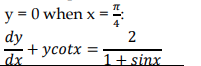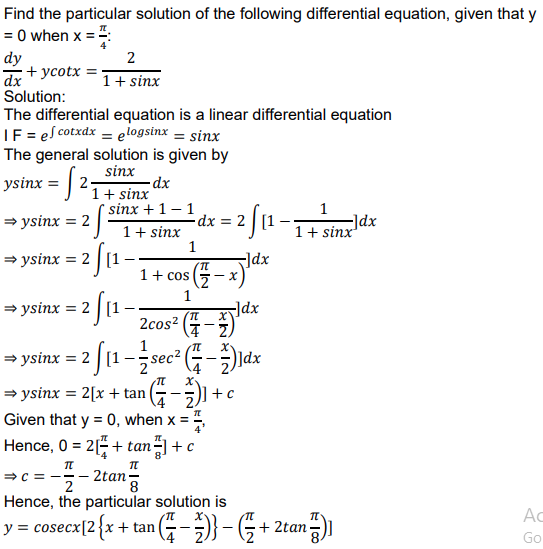# Find the particular solution of the following differential equation, given that

CBSE Sample Question Paper, Class 12 Mathematics Term 2 Question - Find the particular solution of the following differential equation, given that## Find the particular solution of the following differential equation, given that